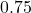## slope point – through:(3,-1) and (-1,-4)

Question

slope point –
through:(3,-1) and (-1,-4)

in progress 0
3 weeks 2021-08-22T18:31:00+00:00 2 Answers 0 views 0

• m = 3/4 or 0.75

Step-by-step explanation:

Use slope formula:

• m = (y₂ – y₁)/(x₂ – x₁)

Substitute values of coordinates:

• m = (-4 – (-1))/(-1 – 3) = -3/-4 = 3/4 or 0.75

the slope of the line is :-

=》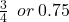Step-by-step explanation:

the slope of the line is :-

=》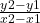=》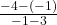=》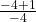=》=》